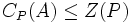# Locally finite Artinian p-group implies hypercentral

Jump to: navigation, search
This article gives the statement and possibly, proof, of an implication relation between two group properties. That is, it states that every group satisfying the first group property (i.e., locally finite Artinian p-group) must also satisfy the second group property (i.e., hypercentral group)
View all group property implications | View all group property non-implications
Get more facts about locally finite Artinian p-group|Get more facts about hypercentral group

## Statement

Any locally finite Artinian P-group (?) is hypercentral: the transfinite Upper central series (?) of the group terminates at the whole group.

## Definition used

### Locally finite group

Further information: Locally finite group

A group is termed locally finite if every finitely generated subgroup of the group is finite.

### Artinian group

Further information: Artinian group

A group is termed Artinian if every nonempty collection of subgroups has a minimal element: a subgroup in that collection that does not contain any other member of that collection.

### p-group

Further information: p-group

A group is termed a p-group for some prime$p$ if the order of every element of the group is a power of the prime$p$.

## Proof

### Reduction

By facts (1), (2), (3), and transfinite induction, it suffices to prove that the center of a nontrivial locally finite Artinian p-group is nontrivial. We do this proof.

### Proof of nontrivial center

Given: A prime$p$, a nontrivial locally finite Artinian$p$-group$P$.

To prove:$Z(P)$ is nontrivial.

Proof:

1. The centralizer of any finitely generated subgroup of$P$ is nontrivial (uses local finiteness): Observe that if the finitely generated subgroup is trivial, then its centralizer is$P$, which by assumption is nontrivial. Otherwise, by the local finiteness condition, the finitely generated subgroup is a nontrivial finite$p$-group, i.e., a group whose order is a power of$p$. By fact (4), this subgroup has a nontrivial center, and thus, its centralizer, which must contain the center, is also nontrivial.
2. The set of centralizers of finitely generated subgroups is closed under finite intersections (uses nothing): Now, let$S$ be the set of centralizers of finitely generated subgroups. Note that if$A$ and$B$ are finitely generated, then so is$\langle A, B \rangle$, and$C_P(\langle A, B \rangle) = C_P(A) \cap C_P(B)$. Thus,$S$ is closed under finite intersections.
3. The set of centralizers of finitely generated subgroups has a member contained in every other member (uses Artinianness): By Artinianness of$P$, there exists a finitely generated subgroup$A$ such that$C_P(A)$ is minimal among elements of$S$. For any finitely generated subgroup$B$,$C_P(A) \cap C_P(B) \le C_P(A)$, and since$S$ is closed under finite intersections, we have a member of$S$ contained in$C_P(A)$. By the minimality of$C_P(A)$, we get$C_P(A) \cap C_P(B) \le C_P(A)$, forcing$C_P(A) \le C_P(B)$ for all finitely generated subgroups$B$.
4. The center is nontrivial: In particular,$C_P(A)$ centralizes every cyclic subgroup of$P$. Thus,$C_P(A)$ centralizes every element of$P$. But this forces$C_P(A) \le Z(P)$. Since$C_P(A)$ is nontrivial, we obtain that$Z(P)$ is nontrivial.